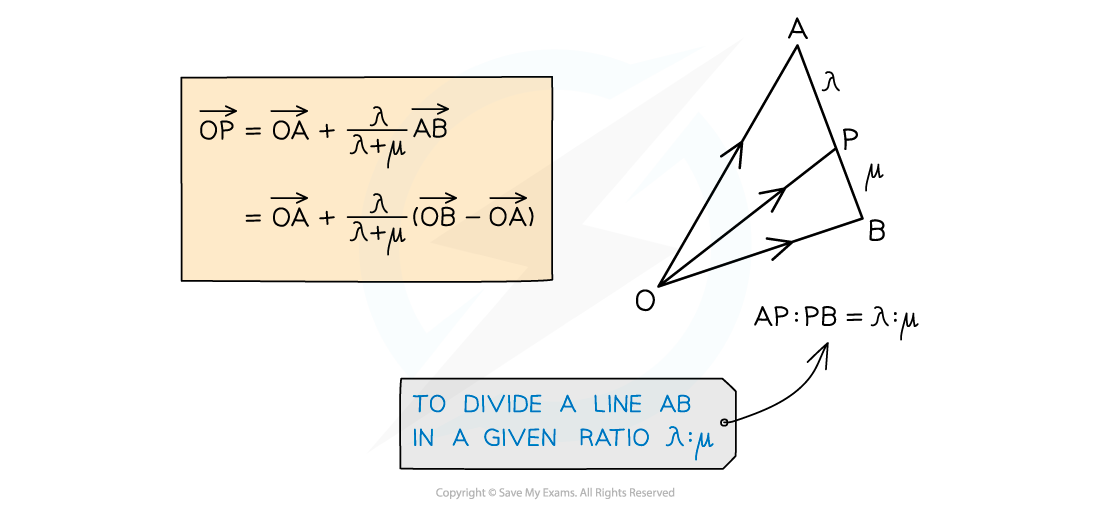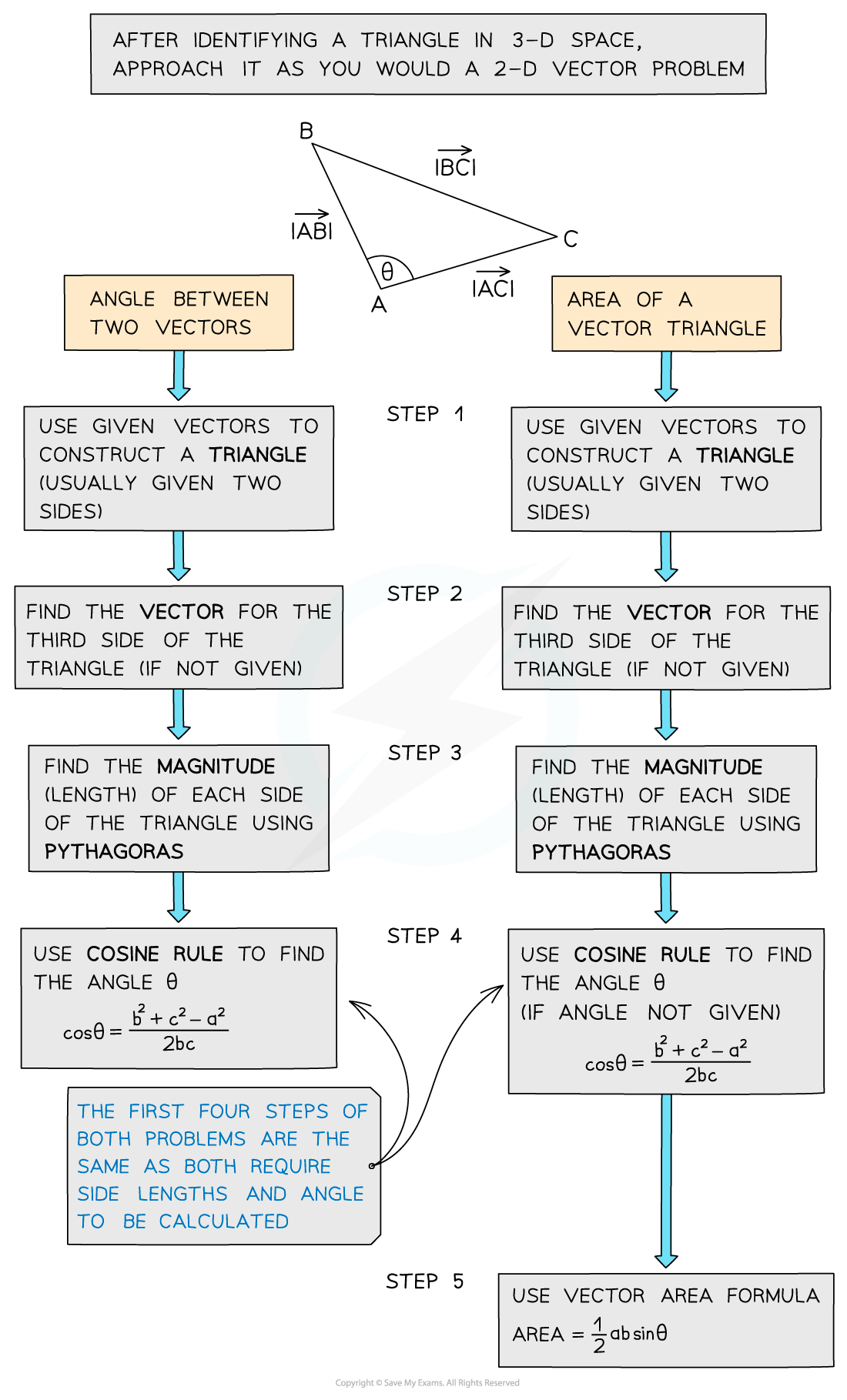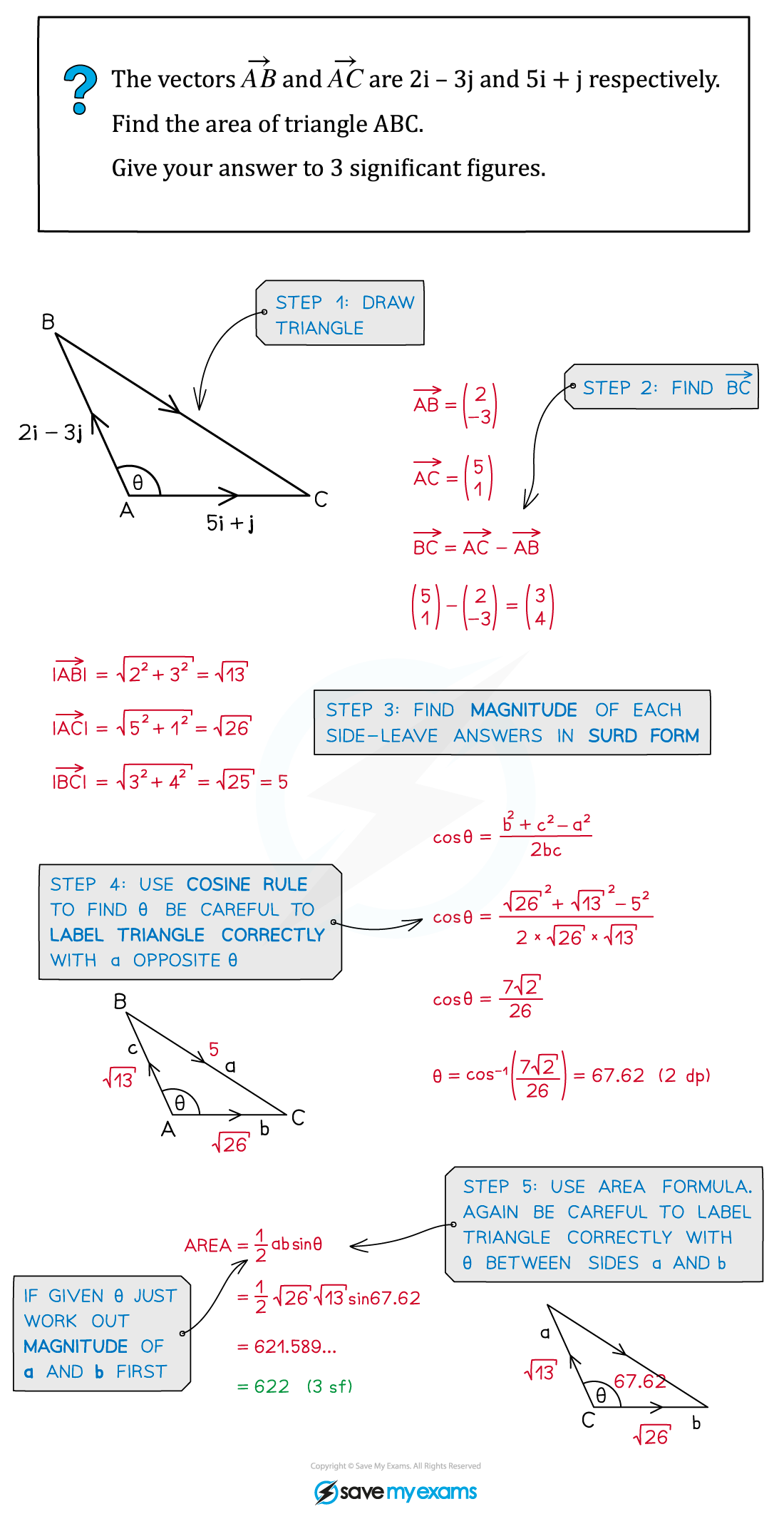# AQA A Level Maths: Pure复习笔记11.1.5 Problem Solving using Vectors

### Problem Solving using Vectors

#### Problem-solving with vectors

• Vectors can be used to prove two lines are parallel (see Vector Addition)
• They can also be used to show points are collinear (lie on the same straight line)
• Vectors can be used to find missing vertices of a given shape
• You will need a good understanding of how to divide a line segment into a given ratio#### Using trig in vector problems

• When problem-solving with vectors, trigonometry can help us:
• convert between component form and magnitude/direction form (see Magnitude Direction)
• find the angle between two vectors using Cosine Rule (see Non-Right-Angled Triangles)
• find the area of a triangle using a variation of Area Formula (see Non-Right-Angled Triangles)#### Exam Tip

• Think of vectors like a journey from one place to another – you may have to take a detour eg. A to B might be A to O then O to B.
• Diagrams can help, if there isn’t one, draw one. For a given diagram labelling all known vectors and quantities will help.

#### Worked Example